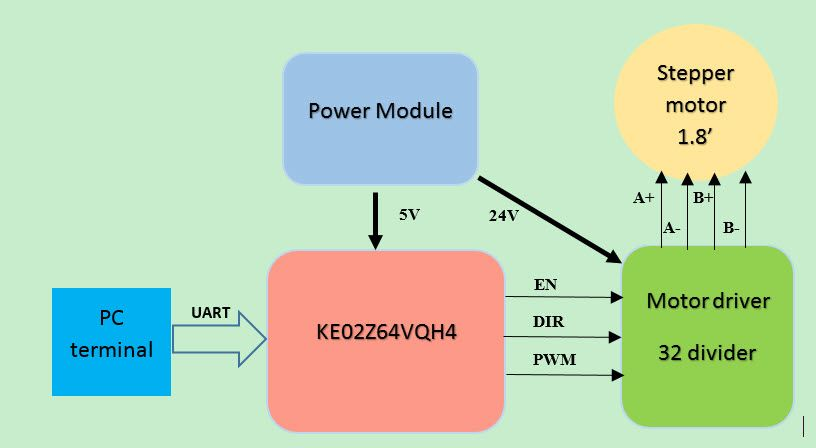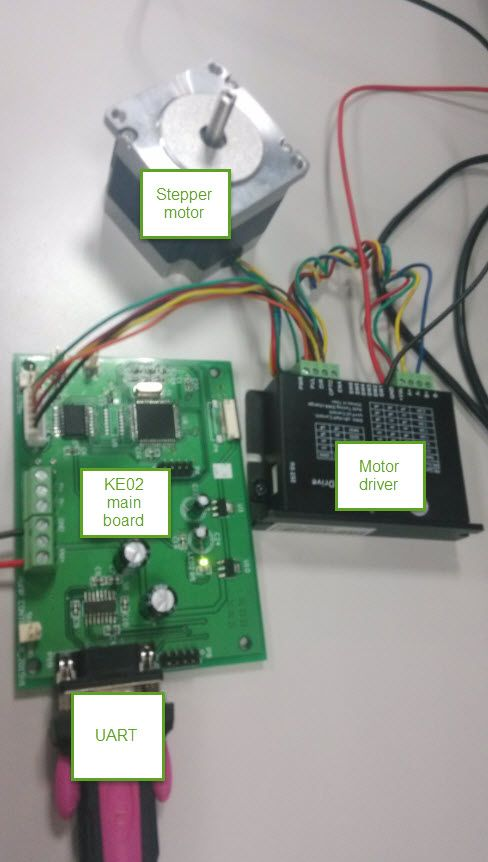# Stepper motor control based on KE02

cancel
Showing results for
Did you mean:

# Stepper motor control based on KE02

No ratings

## Stepper motor control based on KE02

1 Abstract

Stepper motor can be controlled by the electrical pulse signal with the open loop system, it use the electrical pulse signal realize the angular movement or linear movement.  The speed and position of the stepper motor is determined by the pulse frequent and the pulse number. Stepper motor can be used in the low speed area application, with higher work efficiency and low noise.

KE02 is the 5V kinetis E series MCU, it is based on ARM Cortex M0+ core, KE series are designed to maintain high robustness for complex electrical noise environment and high reliability application. For these advantages, KE02 is fit the Stepper motor control application.

This document is mainly about how use the KE02 realize the Stepper motor speed, step and direction control. It can use the UART in the PC to control the Stepper motor speed. The following picture is the control diagram.Fig.1

2. Motor control parameter calculation

Just as Fig.1 shows, KE02 should control the EN, DIR, PWM signal to the motor driver, then realize the stepper motor control. EN is the motor driver enable signal, 0 is enable, 1 is disable; DIR is the stepper motor direction control, 0, clockwise, 1 anticlockwise; PWM is the pulse signal to control the step and speed for the stepper motor.

Stepper motor is 1.8’, it means a round have 360’/1.8’= 200 steps. But because the Motor driver have the divider, it is 32, so one stepper motor round should have 200*32 = 6400 steps.

KE02 system, it use the external 10Mhz crystal, and configure both core and bus frequent to 20Mhz,  it use FTM0 module as the motor pulse generate module, bus clock with 32 prescale used as the FTM0 clock source, choose up counter. If need to change the motor speed and control step, just control the FTM PWM frequent and PWM counter.

For Stepper motor, one FTM period means one motor step. From the reference manual of KE02, we get that, the FTM period in up counting mode is: (MOD-CNTIN+1)*period of the FTM counter clock, if want to change the frequent of motor, just calculate the MOD of FTM is ok, then count the number of the FTM cycle, now assume CNTIN =0, then:

Tftm= (32/20Mhz)*(MOD+1)

From the Stepper Motor and it’s driver, we get that one step time is :

Tmstep= 60/(V*6400)

V is the speed of Motor, the unit is round/minute.

Because Tftm=Tmstep, then we know:

MOD= (60/(V*6400))*(20Mhz/32)-1                     (F1)

In this document, we calculate the speed of 150 round/minute, 110 round/minute, 80 round/minute, 50 round/minute and 0.1 round/minute, according to (F1), we can get the MOD for each speed as the following:

• 150 round/minute   MOD=38
• 110 round/minute   MOD=52
• 80 round/minute     MOD=72
• 50 round/minute     MOD=116
• 0.1 round/minute    MOD=58592

If each speed need to do 10 Stepper motor round, then just control the speed counter number to: 10*6400=64000.

3. MCU pin assignment

PTF0 : DIR

PTF1 : EN

PTA0 : PWM

PTC6 : UART1_RX

PTC7 : UART1_TX

4. code writing

(1)FTM initial code

void STEEPMOTOR_PWM_Init(uint16 MODdata)

{

FTM0_SC = 0;

FTM0_C0SC = 0 ;

FTM0_C0V = 0;

FTM0_C0V = MODdata>>1;

FTM0_MOD=MODdata;

FTM0_SC |=FTM_SC_CLKS(1) | FTM_SC_PS(5) | FTM_SC_TOIE_MASK

enable_irq(17); //enable interrupt

}

MODdata can choose the different Stepper motor speed, eg, 150 round/minute, MODdata is 38.

(2) interrupt service function

void FTM0_IRQHandler(void)

{

FTM0_SC  &= ~FTM_SC_TOF_MASK;//clear the TOF flag.

roundcount++;

if(roundcount >= 64000) {FTM0_C0SC = 0x00; FTM0_SC &= ~(FTM_SC_TOIE_MASK);}

}

It can used for the step counter, and when reach the 10round, then stop the motor( stop the FTM output).

(3)Speed choose with UART input

void Motor_Speed_GPIO_CTRL_30round(void)

{

char motormode=0;

uint32 COMPDATA=0;

printf("\n 1 for 150 round/minute\n\r");

printf("\n 2 for 110 round/minute\n\r");

printf("\n 3 for 80 round/minute\n\r");

printf("\n 4 for 50 round/minute\n\r");

printf("\n 5 for 0.1 round/minute\n\r");

motormode = UART_getchar(PC_TERM_PORT);

switch(motormode)

{

case '1':

STEEPMOTOR_PWM_Init(38);//150 round/minute

break;

case '2':

STEEPMOTOR_PWM_Init(52);//110 round/minute

break;

case '3':

STEEPMOTOR_PWM_Init(72);//80 round/minute

break;

case '4':

STEEPMOTOR_PWM_Init(116);//50 round/minute

break;

case '5':

STEEPMOTOR_PWM_Init(58592);//0.1 round/minute

break;

default: break;

}

while( roundcount < 64000 ) {} //10 round

Disable_PWM;

printf("\n %c 10round PWM is finished ", motormode);

roundcount=0;

}

5 DEMO